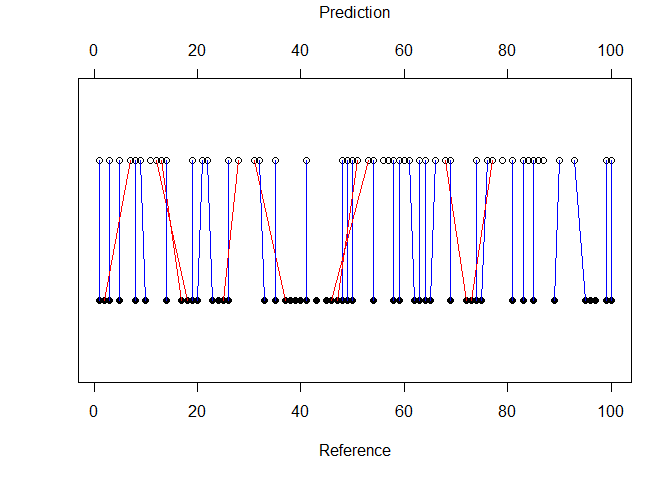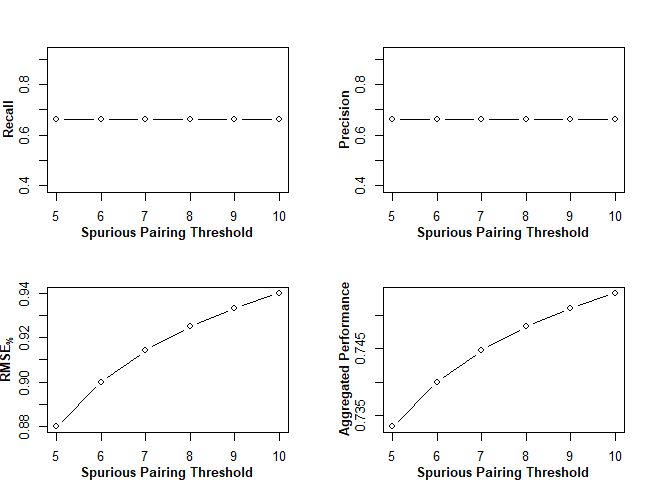# The Transition Pairing Method

## Introduction

This vignette is designed to highlight some basic aspects of using the transition pairing method (TPM) in R. It has been written with the assumption that the reader is already familiar with the TPM itself, and is simply in need of direction on how to implement it. The core functions are PAutilities::get_transition_info and PAutilities::spurious_curve, along with S3 methods for plot and summary, and S4 methods for + and -.

The TPM is used for evaluating dynamic segmentation algorithms, especially in the context of physical activity data from wearable sensors. The best example is an algorithm that aims to identify transitions between different activities. Throughout this vignette, we will suppose we are evaluating such an algorithm.

## Getting Started

Suppose we have two indicator vectors representing the occurrence of a transition (1) or non-transition (0) at certain time points. One vector represents a criterion measure (e.g. direct observation), and the other represents our reference measure (i.e., the aforementioned transition-detection algorithm).

set.seed(100)
algorithm <- (sample(1:100)%%2)
criterion <- (sample(1:100)%%2)

Ideally, the vectors have equal length. If not, the shorter vector will be expanded (with a warning), such that it has the same length as the longer vector, with transitions placed proportionally to the original. For example, suppose we have a 10-item vector with one transition:

{0, 0, 0, 1, 0, 0, 0, 0, 0, 0}

The transition occurs at index 4 of 10, or 40% of the way into the vector. If we expand the vector to size 25, it will become:

{0, 0, 0, 0, 0, 0, 0, 0, 0, 1, 0, 0, 0, 0, 0, 0, 0, 0, 0, 0, 0, 0, 0, 0, 0}

The transition now occurs at index 10 of 25, still 40% of the way into the vector.

## Running the TPM

We will first run the TPM using a spurious pairing threshold of 7.

NOTE: In both get_transition_info and spurious_curve, the spurious pairing threshold values reflect indices, not necessarily seconds. In the example below, the threshold setting (window_size = 7) would reflect seconds if both the predictions and references objects were second-by-second variables. On the other hand, if they were 5-s epochs (i.e., 0.2 Hz sampling rate), window_size = 7 would correspond to a 35-s lag time allowance.

  transitions <- get_transition_info(
predictions = algorithm,
references = criterion,
window_size = 7
)

This gives us an object of class transition. We can first plot it.

  plot(transitions)The visualization can be nice for several purposes, not least of which is seeing which pairings (if any) were made non-sequentially and rejected by the pruning algorithm. (Non-sequential pairings are shown with red pairing lines.)

To obtain performance metrics, we can summarize the transitions object.

  summarized1 <- summary(transitions)

This gives an S4 object of class summaryTransition. The result slot must be accessed to obtain the metrics.

  summarized1@result
#>   window_size reference_positives predicted_positives true_positives
#> 1           7                  50                  50             33
#>   n_rejected_pairs mean_abs_lag_indices sd_abs_lag_indices
#> 1                9            0.3030303          0.5294365
#>   mean_sd_abs_lag_indices mean_signed_lag_indices sd_signed_lag_indices
#> 1               0.3+/-0.5             -0.06060606             0.6092718
#>   mean_sd_signed_lag_indices recall precision rmse_lag_indices rmse_prop
#> 1                -0.06+/-0.6   0.66      0.66              0.6 0.9142857
#>   aggregated_performance
#> 1              0.7447619

# or:
# slot(summarized1, "result")

NOTE: One or more versions prior to PAutilities 1.0.0 included the Rand Index (in various forms) in the output. The associated package is no longer supported, so this feature has been removed. At any rate, it’s not recommendable to use the Rand index, for similar reasons to the Needleman-Wunsch algorithm, as discussed elsewhere. (Background: Essentially the Rand index gives a score between 0 and 1, reflective of how well aligned the criterion and predicted segments are. Different adjustments can be applied, which you can read about in the CLUES paper.)

ANOTHER NOTE: The result slot also includes some extra variables, such as signed lags. Two important variables are rmse_prop and aggregated_performance. The former expresses RMSE in relative terms, i.e., as a value between 0% (pessimal RMSE, equal to the spurious pairing threshold) and 100% (optimal RMSE, equal to 0). The advantage of this metric (RMSE%) is that it puts RMSE on the same scale as recall and precision, allowing all three of them to be averaged into a single indicator of performance, i.e., aggregated_performance. This approach is useful when a single criterion is needed, e.g. for determining which algorithm settings provide the best performance.

## Moving On

At this point, the TPM process may seem unnecessarily complicated:

• First use get_transition_info to obtain a transition object.
• Then run summary on the object.
• Then access the result slot.

Even with magrittr pipes, this takes up some space:

  suppressPackageStartupMessages(
library(magrittr, quietly = TRUE, verbose = FALSE)
)

summarized <-
get_transition_info(algorithm, criterion, 7) %>%
summary(.) %>%
slot("result")

Here’s why that level of separation is worthwhile: It makes it possible to combine objects or look at how different they are. Let’s say we ran our algorithm on two separate occasions.

  # Here I'm exploiting seed changes to get different values from the same code
# I used previously

algorithm2 <- (sample(1:100)%%2)
criterion2 <- (sample(1:100)%%2)

We may be interested in looking at the combined performance for both occasions. For that, we can add summary objects together.

  summarized2 <-
get_transition_info(algorithm2, criterion2, 7) %>%
summary(.)

# Store the result of addition (another S4 summaryTransition object)

# Now view the result

#>   window_size reference_positives predicted_positives true_positives
#> 1           7                 100                 100             71
#>   n_rejected_pairs mean_abs_lag_indices sd_abs_lag_indices
#> 1               16            0.3098592          0.5234924
#>   mean_sd_abs_lag_indices mean_signed_lag_indices sd_signed_lag_indices
#> 1               0.3+/-0.5                       0             0.6094494
#>   mean_sd_signed_lag_indices recall precision rmse_lag_indices rmse_prop
#> 1                  0.0+/-0.6   0.71      0.71              0.6 0.9142857
#>   aggregated_performance
#> 1              0.7780952

# or:
# slot(added, "result")

Or we may be interested in looking at whether the algorithm performed better on one occasion than the other. For that, we can subtract. (NOTE: The subtraction method returns a list, not an S4 object, and the differences element is the item of interest.)

  subtracted <- summarized1 - summarized2

subtracted\$differences
#>   window_size diff_window_size diff_recall diff_precision
#> 1           7                0        -0.1           -0.1
#>   diff_mean_abs_lag_indices diff_mean_signed_lag_indices
#> 1               -0.01275917                   -0.1132376
#>   diff_rmse_lag_indices diff_rmse_prop diff_aggregated_performance
#> 1                     0              0                 -0.06666667

## Running a spurious curve analysis

It’s useful to run your analysis for multiple values of the spurious pairing threshold. That can also be done conveniently through PAutilities. Let’s look at how performance changes (for our original transitions object, obtained when we first ran the algorithm) when we use settings of 5-10.

  curve <- spurious_curve(trans = transitions, thresholds = 5:10)
class(curve)
#>  "list"           "spurious_curve"
sapply(curve, class)
#>  "summaryTransition" "summaryTransition" "summaryTransition"
#>  "summaryTransition" "summaryTransition" "summaryTransition"

That gives us a list of summaryTransition objects for each threshold setting. The list also inherits class spurious_curve, which has a convenient plot method.

  par(mar=rep(3,4))
plot(curve)## Conclusion

This vignette has provided a crash course in running the TPM in different ways. Setting up a full analysis can still take some work, but PAutilities provides solid infrastructure to help you do so in a controlled way. Suggested improvements are welcome. You can post issues or pull requests on the package GitHub site. See you there.